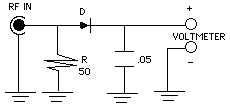RF WATTMETER

Cost estimate: \$2 (excluding meter)

This useful project is so simple I didn't include a photo! With a few components, a voltmeter and a little math you can measure the approximate output of your QRP transmitter.

Resistor R is the load (a dummy load: 50 ohms). RF voltage is sampled across the load and rectified; DC voltage appears at the meter terminals. Make sure the total power rating of R can handle your transmitter's anticipated output. For the "Michigan Mighty Mite" use two 1/2-watt 100-ohm resistors in parallel, which yields a 1-watt power rating: plenty enough for that transmitter. A ceramic disc type capacitor is fine; use a germanium (1N34A) diode or Schottky diode such as a 1N5711. A small-signal silicon "glass" diode such as the 1N914 will work, but add a little less than avolt to the readout before computing to compensate for its voltage drop. (The voltage drop for a germanium diode is about 0.3V; for a silicon diode about 0.7V)

```To determine approximate peak power output in watts:

Power = (ExE)/R

where

E =  Meter reading in volts

and

R =  Load Resistance in ohms (50 ohms)

```
When powered by 14 volts my version of the Mighty Mite makes the meter read 4.5 volts, so
```                     Power= (4.5 x 4.5) / 50
= 20.25 / 50
= 0.405 W
...is the approximate peak power output.```
I wouldn't bet on the absolute accuracy of this, but it does give an in-the-ballpark indication of what's being transmitted.

```Source: